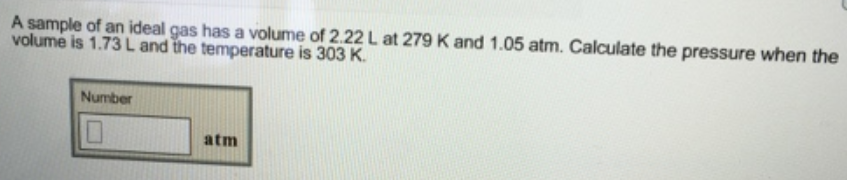# Problem:  A sample of an ideal gas has a volume of 2.22 L at 279 K and 1.05 atm. Calculate the pressure when the volume is 1.73 L and the temperature is 303 K.

###### FREE Expert Solution
99% (237 ratings)###### Problem Details

A sample of an ideal gas has a volume of 2.22 L at 279 K and 1.05 atm. Calculate the pressure when the volume is 1.73 L and the temperature is 303 K.